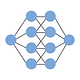# Build your first Convolutional Neural Network to recognize images

## A step-by-step guide to building your own image recognition software with Convolutional Neural Networks using Keras on CIFAR-10 images!

You can write your own image recognition software with just a few lines of code! In this post, we will see how to use Keras to build Convolutional Neural Networks to predict what’s inside a small image. We will go through the full Deep Learning pipeline, from:

• Exploring and Processing the Data
• Building and Training our Convolutional Neural Network
• Testing out with your own images

Pre-requisites:

This post assumes you’ve had extremely basic experience with using Keras to build a standard neural network. If you have not done so, please follow the instructions in the tutorial below:

This is a Coding Companion to Intuitive Deep Learning Part 2. As such, we also assume that you have some intuitive understanding of Convolutional Neural Networks. If you need a refresher, please read the intuitive introduction below:

Resources you need:

Optionally, you may download an annotated Jupyter notebook which has all the code covered in this post here.

And now, let’s begin!

# Exploring and Processing the Data

In this section, we’ll do the following:

• Change the label to one-hot encodings
• Scale the image pixel values to take between 0 and 1

Let’s begin! To do image recognition, we will first need a dataset and the labels of what is contained in the image. Thankfully, we don’t have to manually scrape the web for images and label them ourselves, as there are a few standard datasets that we can play around with.

In this post, we will use the CIFAR-10 dataset. The details of the dataset are as follows:

• Images to be recognized: Tiny images of 32 * 32 pixels
• Labels: 10 possible labels (airplane, automobile, bird, cat, deer, dog, frog, horse, ship, and truck)
• Dataset size: 60000 images, split into 50000 for training and 10000 for testing

Here is an example of the images and their labels: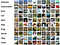Images and their labels from the CIFAR-10 dataset. Image taken from https://www.cs.toronto.edu/~kriz/cifar.html

The first thing we need to do is to get the image dataset. We do so with Keras as well, by running the following code in our Jupyter notebook:

`from keras.datasets import cifar10(x_train, y_train), (x_test, y_test) = cifar10.load_data()`

Now, the data that we need are stored in the respective arrays (x_train, y_train, x_test and y_test). Let us explore the dataset a little.

Let’s see what the shape of our input features array is:

`print('x_train shape:', x_train.shape)`

You should see something like this:

The shape of the array tells us that our dataset x_train consists of:

• 50000 images
• 32 pixels in height
• 32 pixels in width
• 3 pixels in depth (corresponding to Red, Green and Blue)

Let’s see what the shape of the label array is:

`print('y_train shape:', y_train.shape)`

You should get the following output:

This means that there is one number (corresponding to the label) for each of out 50000 images.

Now, let’s try to see an example of an image and a label to make things concrete. If we try to print the very first image like this:

`print(x_train)`

You should see a series of numbers:

While that’s how the computer sees the image, that isn’t terribly helpful for us. So let’s visualize this image of x_train using the matplotlib package:

`import matplotlib.pyplot as plt%matplotlib inlineimg = plt.imshow(x_train)`

The `%matplotlib inline` tells the notebook that you wish for the image to display within the image. `plt.imshow` is a function that displays the numbered pixel values in `x_train`to the actual image it represents. You should see Jupyter notebook display a picture for you:

It is pretty pixelated, but that’s because the size of the image is 32 * 32 pixels, which is extremely tiny! Let’s figure out what the label of this image is in our dataset:

`print('The label is:', y_train)`

You should get something like this:

We see that the label is the number ‘6’. The conversion of numbers to the label is alphabetically sorted as follows:

So, from the table we can see that the above image was labelled as a picture of a frog (label: 6). Let’s try seeing another example of an image, by changing the index to 1 (2nd image in our dataset) instead of 0 (1st image in our dataset):

`img = plt.imshow(x_train)`

We can display its label as well:

`print('The label is:', y_train)`

The image and its corresponding label should look like this:

Using the table we used earlier, we see that this image is labelled as a truck.

Now that we’ve explored our dataset, we need to process it.

The first observation we make is that our labels as the class number isn’t very helpful. This is because the classes are not ordered in any way. Let’s give an example to illustrate this. What if our neural network thinks it can’t decide whether the image is an automobile (label: 1) or a truck (label: 9). Should we take the halfway point and predict it as a dog (label: 5)? That barely makes any sense.

For those of you who read the previous post, Build your first Neural Network to predict house prices with Keras, you might wonder why we could use the labels  and  there. That’s because there are only two classes, and we can interpret the output of the neural network as a probability. That is, if the neural network outputs 0.6, it means it believes it is above median house price with 60% probability. This doesn’t work in a multi-class setting like this, where the image can belong to one of 10 different classes.

What we really want is the probability of each of the 10 different classes. For that, we need 10 output neurons in our neural network. Since we have 10 output neurons, our labels must match this as well.

To do this, we convert the label into a set of 10 numbers where each number represents if the image belongs to that class or not. So if an image belongs to the first class, the first number of this set will be a 1 and all other numbers in this set will be a 0. This is called a one-hot encoding, and the conversion table now looks like this:

To do this conversion in code, we use Keras again:

`import kerasy_train_one_hot = keras.utils.to_categorical(y_train, 10)y_test_one_hot = keras.utils.to_categorical(y_test, 10)`

The line `y_train_one_hot = keras.utils.to_categorical(y_train, 10)`means that we take the initial array with just the number, y_train, and convert it to the one_hot encodings, y_train_one_hot. The number 10 is required as a parameter as you need to tell the function how many classes there are (10).

Now, let’s say we want to see how the label for our second image (the truck, label: 9) looks like in the one-hot setting:

`print('The one hot label is:', y_train_one_hot)`

Your Jupyter notebook should look like this: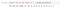One-hot label for our second image, which is an image of a truck (label: 9)

Now that we’ve processed our labels (y), we might also want to process our image (x). A common step we do is to let the values to be between 0 and 1, which will aid in the training of our neural network. Since our pixel values already take the values between 0 and 255, we simply need to divide by 255.

`x_train = x_train.astype('float32')x_test = x_test.astype('float32')x_train = x_train / 255x_test = x_test / 255`

In practice, what we do is convert the type to ‘float32', which is a datatype that can store values with decimal points. Then, we divide each cell by 255. If you want, look at the array values of the first training image by running the cell:

`x_train`

You should see your Jupyter notebook like this: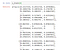The array values of our first image that has been normalized to be between 0 and 1

Now, notice that so far we’ve only had a train set and a test set. Unlike our previous post, we will not pre-split our validation set as there is a shortcut for this that we’ll introduce later on.

Summary: In exploring and processing the data, we’ve:

• Changed the label to one-hot encodings
• Scale the image pixel values to take between 0 and 1

# Building and Training our Convolutional Neural Network

Similar to our previous post, we need to define the architecture (template) first before fitting the best numbers into this architecture by learning from the data.

First Step: Setting up the Architecture

The CNN architecture that we will build is as follows:

Wow! That is a lot of layers (more than we’ve seen so far), but all built from concepts we’ve seen before. Each layer will later correspond to roughly one line of code only, so persevere on because things will shape up really soon.

Recall that the last layer (the softmax layer) simply transforms the output of the previous layer into probability distributions, which is what we want for our classification problem.

If you recall from Intuitive Deep Learning Part 2, there is one thing we have not specified above, which is padding. For now, we’ll zero-pad our layer such that the output width and height will be the same as the input width and height. This is called ‘same’ padding. For a 3x3 filter, to achieve the same width and height, we’ll have to pad with a border width of 1. We will be applying ‘same’ padding for all the conv layers.

Lastly, we will use ReLU activation for all our layers, except for the last layer which is a softmax activation. Now, it’s time to code!

To code this in, we’ll use Keras sequential model. However, since we have many layers in our model, I’ll introduce a new way to specify the sequence. We’ll go through the code line-by-line, so that you can follow what we are doing exactly. First, we import some of the code we need:

`from keras.models import Sequentialfrom keras.layers import Dense, Dropout, Flatten, Conv2D, MaxPooling2D`

Then, we call an ‘empty’ sequential model:

`model = Sequential()`

We’ll add to this empty model one layer at a time. The first layer (if you recall from our diagram) is a conv layer with filter size 3x3, stride size 1 (in both dimensions), and depth 32. The padding is the ‘same’ and the activation is ‘relu’ (these two settings will apply to all layers in our CNN). With all that, let’s specify our first layer in code:

`model.add(Conv2D(32, (3, 3), activation='relu', padding='same', input_shape=(32,32,3)))`

What this is doing is to add this layer to our empty sequential model using the function `model.add()`. The first number 32 refers to the depth. The next pair of numbers (3,3) refer to the filter width and size. Then, we specify activation which is ‘relu’ and padding which is ‘same’. Notice that we did not specify stride. This is because stride=1 is a default setting, and unless we want to change this setting, we need not specify it.

If you recall, we also need to specify an input size for our first layer; subsequent layers does not have this specification since they can infer the input size from the output size of the previous layer.

Our second layer looks like this in code (we don’t need to specify the input size):

`model.add(Conv2D(32, (3, 3), activation='relu', padding='same'))`

The next layer is a max pooling layer with pool size 2 x 2 and stride 2 (in both dimensions). The default for a max pooling layer stride is the pool size, so we don’t have to specify the stride:

`model.add(MaxPooling2D(pool_size=(2, 2)))`

Lastly, we add a dropout layer with probability 0.25 of dropout so as to prevent overfitting:

`model.add(Dropout(0.25))`

And there we have it, our first four layers in code. The next four layers look really similar (except the depth of the conv layer is 64 instead of 32):

`model.add(Conv2D(64, (3, 3), activation='relu', padding='same'))model.add(Conv2D(64, (3, 3), activation='relu', padding='same'))model.add(MaxPooling2D(pool_size=(2, 2)))model.add(Dropout(0.25))`

Lastly, we have to code in our fully connected layer, which is similar to what we’ve done in our previous post, Build your first Neural Network. However, at this point, our neurons are spatially arranged in a cube-like format rather than in just one row. To make this cube-like format of neurons into one row, we have to first flatten it. We do so by adding a Flatten layer:

`model.add(Flatten())`

Now, we have a dense (FC) layer of 512 neurons with relu activation:

`model.add(Dense(512, activation='relu'))`

We add another dropout of probability 0.5:

`model.add(Dropout(0.5))`

And lastly, we have a dense (FC) layer with 10 neurons and softmax activation:

`model.add(Dense(10, activation='softmax'))`

And we’re done with specifying our architecture! To see a summary of the full architecture, we run the code:

`model.summary()`

The Jupyter notebook should look like this at this point:

Second Step: Filling in the best numbers

We’ll compile the model with our settings below:

`model.compile(loss='categorical_crossentropy',              optimizer='adam',              metrics=['accuracy'])`

The loss function we use is called categorical cross entropy, which is applicable for a classification problem of many classes. The optimizer we use here is Adam. We haven’t gone through the intuition of Adam yet, but know that Adam is simply a type of stochastic gradient descent (with a few modifications) so that it trains better. Lastly, we want to track the accuracy of our model.

And now, it’s time to run our training:

`hist = model.fit(x_train, y_train_one_hot,            batch_size=32, epochs=20,            validation_split=0.2)`

We train our model with batch size 32 and 20 epochs. However, do you notice something different about the code? We use the setting `validation_split=0.2` instead of `validation_data`. With this shortcut, we did not need to split our dataset into a train and validation set at the start! Instead, we simply specify how much of our dataset will be used as a validation set. In this case, 20% of our dataset is used as a validation set.

Run the cell and you’ll see the model starts training. This model takes a lot longer to train on your CPU, so you might have to go get a cup of coffee before everything is done.

After you’ve done training, we can visualize the model training and validation loss over the number of epochs using this code we’ve seen in Build your first Neural Network:

`plt.plot(hist.history['loss'])plt.plot(hist.history['val_loss'])plt.title('Model loss')plt.ylabel('Loss')plt.xlabel('Epoch')plt.legend(['Train', 'Val'], loc='upper right')plt.show()`

Your graph might look something like this:

We can also visualize the accuracy:

`plt.plot(hist.history['acc'])plt.plot(hist.history['val_acc'])plt.title('Model accuracy')plt.ylabel('Accuracy')plt.xlabel('Epoch')plt.legend(['Train', 'Val'], loc='lower right')plt.show()`

Your graph might look something like this:

At this point, I encourage you to go back and try out different hyper-parameters such as changing the architecture or increasing the number of epochs to see if you can get a better val accuracy. And once you’re happy with your model, you can evaluate it on the test set:

`model.evaluate(x_test, y_test_one_hot)`

I got an accuracy like this:

This is a lot better than if our model guessed at random (10% accuracy), although not quite human accuracy yet (Human accuracy is roughly 94%). There are still some things that can be improved from our vanilla CNN we have here, and we’ll cover some of these improvements in more advanced topics.

At this point, you might want to save your trained model (since you’ve spent so long waiting for it to train). The model will be saved in a file format called HDF5 (with the extension .h5). We save our model with this line of code:

`model.save('my_cifar10_model.h5')`

You should see the saved model in the directory of your notebook. If you ever want to load your saved model in the future, use this line of code:

`from keras.models import load_modelmodel = load_model('my_cifar10_model.h5')`

We won’t do the above line in this notebook, but it’s for your reference and will be useful when we are deploying our model online.

Summary: We’ve built our very first CNN to create an image classifier. In doing so, we’ve used the Keras Sequential model to specify the architecture, and trained it on the dataset we’ve pre-processed earlier. We’ve also saved our model so that we can use it to do image classification later without having to train the model all over again.

# Testing out with your own images

Now that we have a model, let’s try it on our own images. To do so, place your image in the same directory as your notebook. For the purposes of this post, I’m going to use this image of a cat (which you can download here):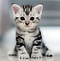Our own cat image that we are going to test our model on

My image file is ‘cat.jpg’. Now, we read in our JPEG file as an array of pixel values:

`my_image = plt.imread("cat.jpg")`

The first thing we have to do is to resize the image of our cat so that we can fit it into our model (input size of 32 * 32 * 3). Instead of coding a resize function ourselves, let’s download a package called ‘scikit-image’ which would help us with that function.

If you need a refresher on Anaconda and installing environment packages, please refer to the tutorial here:

Once you’ve done that, we can go back to our Jupyter notebook and start using the functions we need.

`from skimage.transform import resizemy_image_resized = resize(my_image, (32,32,3))`

We can visualize our resized image like this:

`img = plt.imshow(my_image_resized)`

If you’ve used the same image I did, your Jupyter notebook should output something like this: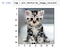A more pixelized version of the cat picture as it has been resized to 32 * 32 * 3 to fit the input shape of our model.

Note that the resized image has pixel values already scaled between 0 and 1, so we need not apply the pre-processing steps that we previously did for our training image. And now, we see what our trained model will output when given an image of our cat, using the code of `model.predict`:

`import numpy as npprobabilities = model.predict(np.array( [my_image_resized,] ))`

This might look confusing, but `model.predict` expects a 4-D array instead of a 3-D array (with the missing dimension being the number of training examples). This is consistent with the training set and test set that we had previously. Thus, the `np.array(...)` code is there to change our current array of `my_image_resized` into a 4-D array before applying the `model.predict` function.

The outputs of the code above are the 10 output neurons corresponding to a probability distribution over the classes. If we run the cell

`probabilities`

we should be able to see the probability predictions for all the classes:

To make our model predictions easier to read, run the code snippet below:

`number_to_class = ['airplane', 'automobile', 'bird', 'cat', 'deer', 'dog', 'frog', 'horse', 'ship', 'truck']index = np.argsort(probabilities[0,:])print("Most likely class:", number_to_class[index], "-- Probability:", probabilities[0,index])print("Second most likely class:", number_to_class[index], "-- Probability:", probabilities[0,index])print("Third most likely class:", number_to_class[index], "-- Probability:", probabilities[0,index])print("Fourth most likely class:", number_to_class[index], "-- Probability:", probabilities[0,index])print("Fifth most likely class:", number_to_class[index], "-- Probability:", probabilities[0,index])`

Your Jupyter notebook should now look like this:

As you can see, the model has accurately predicted that this is indeed an image of a cat. Now, this isn’t the best model we have and accuracy has been quite low, so don’t expect too much out of it. This post has covered the very fundamentals of CNNs on a very simple dataset; we’ll cover how to build state-of-the-art models in future posts. Nevertheless, you should be able to get some pretty cool results from your own images.

Consolidated Summary: In this post, we’ve written Python code to:

• Explore and Process the Data
• Build and Train our first CNN and save the model
• Test our image classifier on our own images

Congratulations! You’ve built your very first Deep Learning image classifier. As before, we haven’t written too many lines of code in this Computer Vision program, and that is because people have written tons of code so that all we needed to do was to plug-and-play the relevant functions they’ve written.

What’s Next: At this point you might be wondering: we’ve built a working image classifier, how do I show it to others and test it on a platform that is not Jupyter notebook? If you wish to build a web application around your Machine Learning model so that others can try it out too, this optional coding post will show you how:

In our next Coding Companion Part 3 (link to be released), we will explore how to code up our own Recurrent Neural Networks (RNNs) to deal with natural language! Be sure to get an intuitive understanding of RNNs here:

Intuitive Deep Learning Part 3: RNNs for Natural Language Processing

Written by

Written by

## Joseph Lee Wei En

#### I want to make Deep Learning concepts as intuitive and as easily understandable as possible by everyone! Follow my publication to learn more!